MORE IN Engineering Mechanics
MU First Year Engineering (Semester 1)
Engineering Mechanics
May 2014
Total marks: --
Total time: --
INSTRUCTIONS
(1) Assume appropriate data and state your reasons
(2) Marks are given to the right of every question
(3) Draw neat diagrams wherever necessary

1 (a) Two concurrent forces P and Q acts at O such that resultant acts along x-axis. Determine the magnitude of Q and hence the resultant.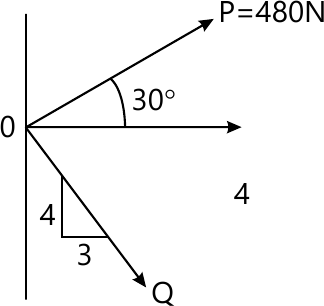4 M
1 (b) A cylinder with 1500 N weight is resting in an unsymmetrical smooth groove as shown in figure. Determine the reactions at the point of contacts.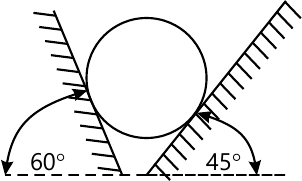4 M
1 (c) Expiain Angle of Friction, Angle of Repose and the relation between the two.
4 M
1 (d) A sprinter in a 100m race accelerates uniformly for the first 35m and then runs with constant velocity. If the sprinter's time for the first 35m is 5.4 seconds, determine his time for the race.
4 M
1 (e) A motorist travelling at a speed of 90kmph suddenly applies the brackes and comes to rest after skidding 100m. Determine the time required for the car to stop and coefficient of kinetic friction between the tires and the road.
4 M

2 (a) A system of forces acting on a bell crank is as shown. Determine the magnitude, direction and the point of application of the resultant w.r.t. 'O'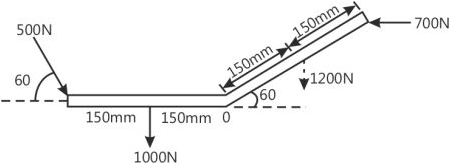6 M
2 (b) Two cylinder are kept in a channel as shown in figure. Determine the reactions at all the contact points A,B,C and D. Assume all surfaces smooth.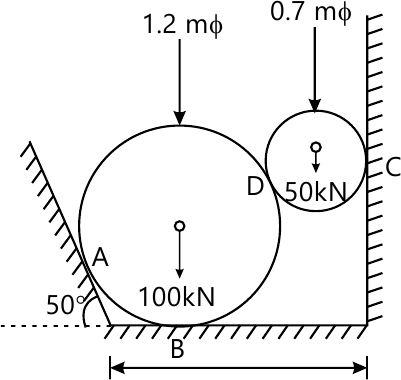8 M
2 (c) A ball of mass 'm' hits directly to a similar ball of mass 'm' which is at rest. The velocity of first ball after impact is zero. Half of the initial kinetic energy is lost in impact. Find coefficient of restitution.
6 M

3 (a) Determine the Centre of gravity of the shadded area.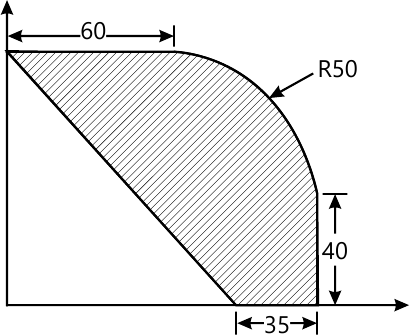8 M
3 (b) The lines of action of three forces concurrent at origin 'O' pass respectively through points A(-1,2,4), B(3,0,-3) and C(2,-2,4)m. The magnitude of forces are 40N, 10N and 30N respectively. Determine the magnitude and direction of their resultant.
6 M
3 (c) A 30N block is released from rest. It slides drown a rough incline having coefficient of friction 0.25. determine the maximum compression of the spring.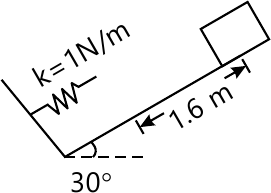6 M

4 (a) Find the support reaction at B and the load P, for the beam shown in figure if the reaction at support A is zero.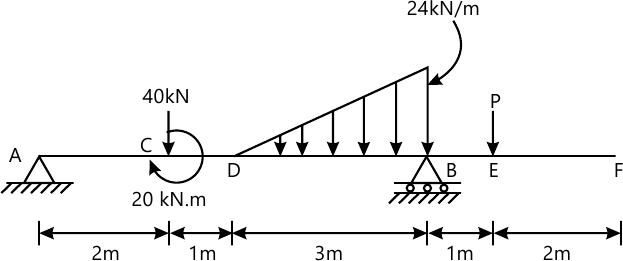8 M
4 (b) A gunman fires a bullet with a velocity of 100m/s 50? upwards from the top of a bill 300m high to hit a bird. The bullet misses its target and finally lands on the ground. Calculate (a) the maximum height reached by the bullet above the ground (b) total time of flight (c) velocity with which the bullets hits the ground.
6 M
4 (c) In the mechanism shown the angular velocity of link AB is 5 rad/sec anticlockwise. At the instant shown, determine the angular velocity of link BC velocity of piston C.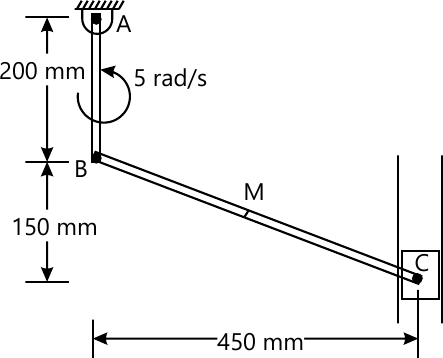6 M

5 (a) Determine the forces in members BC, CE and DE by method of sections and all other members by method of joints. Give the result in a table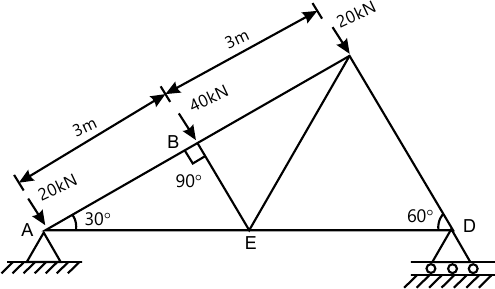8 M
5 (b) Due to slipping points A and B on the rim of the disk have the velocity as shown in figure. Determine the velocity of the centre point C and point D on the rim at this instant. Take radius of disk 0.24m.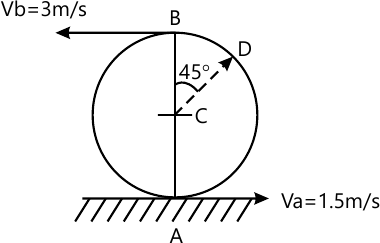6 M
5 (c) A particle moves in a straight line with a velocity-time diagrams shown in figure. If S=-25m at t=0, draw displacement time and accelerator time diagrams for 0 to 24 seconds.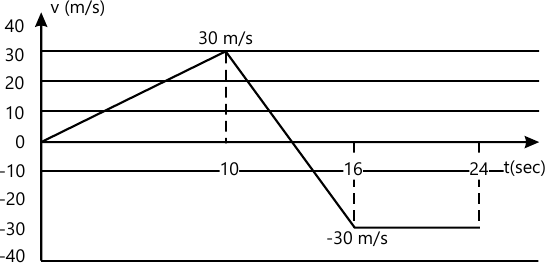6 M

6 (a) The mass of A is 23kg and mass of B is 36kg. The coefficient of friction are 0.4 between A and B, and 0.2 between ground and block B. Assume smooth drum. Determine the maximum mass of M at impending motion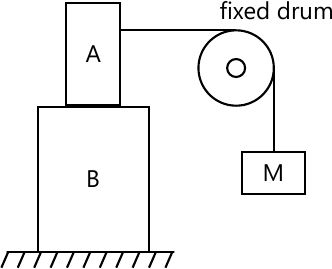8 M
6 (b) A force of 1200N acts along PQ, P(4,5,-2) and Q(-3,1,6)m. calculate its moment about a point A(3,2,0)m
4 M
6 (c) A point moves along the path y=x2/3 with a constant speed of 8m/s. What are the x and y component of the velocities when x=3. What is the acceleration of the point when x=3.
4 M
6 (d) A two step pulley support two weights A=40N and B=60N as shown. Find the downward acceleration of A if radius of bigger pulley is double that of the smaller one. Neglect friction and inertia of pulley.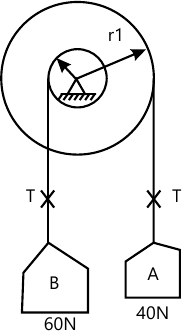4 M

More question papers from Engineering Mechanics